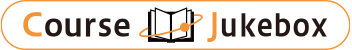### CourseDetailDegree
Master
1, 2
Course delivery methods
face-to-face
Subject
Physical sciences, Mathematical sciences
Program
School
Master's Program in Engineering Sciences
Department
Master's Program in Engineering Sciences
Campus
Tsukuba Campus
Classroom
3A408
Course Offering Year
2023-2024
Course Offering Month
October - November
Weekday and Period
Thu4,5
Capacity
Credits
1.0
Language
English
Course Number
0AJG021

### Statistical Mechanics I University of Tsukuba

#### Course Overview

This lecture begins with a review of basic concepts of statistical mechanics (partition functions, black body radiation, specific of crystal, ideal quantum gas, Fermi-Dirac distribution, Bose-Einstein distribution, etc.), followed by the formulation of statistical mechanics in terms of the density matrix which is an essential tool for dealing with quantum-mechanical many-body systems. Then, we discuss the Wigner function and the perturbation expansion.

#### Learning Achievement

Confirming the basic concept of statistical mechanics, and understanding the formulation to statistical mechanics by density matrix description

#### Competence

This course corresponds to the educational objectives basic ability required to be an independent researcher or engineer and basic ability essential to practical engineering.

#### Course prerequisites

Knowledge of the basics of quantum and statistical mechanics.

Grades will be determined on the basis of assignments and occasional quizzes given during the classes.

#### Course schedule

This lecture begins with a review of basic concepts of statistical mechanics (partition functions, black body radiation, specific heat of crystal, ideal quantum gases, Fermi-Dirac distribution, Bose-Einstein distribution, etc.), followed by the formulation of statistical mechanics in terms of the density matrix which is an essential tool for dealing with quantum-mechanical many-body systems. Then, we discuss the Wigner function and the perturbation expansion.(course schedule)
1. Basic statical mechanics (1): distribution function
2. Basic statical mechanics (2): blackbody radiation, lattice vibration
3. Basic statical mechanics (3): specific heat, Mossbauer effect
4. Basic statical mechanics (4): quantum statistical mechanics in many body system
5. Basic statical mechanics (5): Bose gas and Fermi gas
6. Density matrix (1): basic of the density matrix
7. Density matrix (2): the density matrix in statical mechanics
8. Density matrix (3): examples of the density matrix
9. Density matrix (4): Wigner function, symmetrized density matrix, partial density matrix
10. Density matrix (5): perturbation theory of density matrix

Lectures